Posted onThere is no wrong way to grade. It’s completely up to you. You are in charge.

But sometimes we need some help deciding where to even begin. Our advice is to remember that the goal is learning, not grades. Grades are a reflection to OTHERS of how much you’ve learned. Most people can game this system and make great grades while knowing very little. This is especially true in math. Students are great at crunching the mechanics of math and can make the grade, but then actually have little understanding.

The ACT/SAT exams are geared to catch those deficiencies. They test for understanding rather than crunching. On those exams there isn’t enough time to crunch out the answers, there is a few seconds to guess the correct answer based on your understanding and elimination of impossible answers.

Math is critical thinking. Logic.

Learning and understanding is your goal.  At this point, your child is in high school – young adults. If they aren’t already, its time for them to own their education. Let them check their work. Help them understand, if they cheat, they are only cheating themselves.

Below are some options to choose from for grading. Using these methods, you may discover a combination of them or a completely different method works best for you. But here’s some ideas to start from:

Option 1:

With our children, we did not give a grade for daily work. We did have them check their work and redo the problems they missed.  The key here is to have the STUDENT check their OWN work. Then have them rework the missed problems – this is where learning happens!

Option 2:

Have the student check and rework their problems and give some credit, not for correct problems but, for the work being done as a percentage of their total grade.

Example:

30% of the total grade for homework (100% of this if they do and check all their problems)

70% of the total grade for tests

This option takes some pressure off the tests and incentive to do homework, however, if you follow our test grading guide that gives points back for corrections (again, where real learning happens), then the tests aren’t too pressured anyway.

We also give our tests as open book tests. Math books are good resources and everything in there can’t be memorized as you continue on into higher math. You will need the resource. If you are not comfortable with open-book testing, you could allow them a “cheat sheet” or “note sheet” where they put down the formulas, theorems, and anything from the sections the test is on to use during the test. Even in college math, we were allowed a cheat sheet of a certain size (usually a large notecard or half 8.5×11 paper) for formulas or anything we wanted to put on it.

The goal is to know how to use all the math tools, not to keep the toolbox in your head with no understanding of what they do.

In our AskDrCallahan Teacher’s Guide, you will find a test grading guide (mentioned above) that allows for regaining points for reworked problems.

Example:

Attempt # 1

a)  Number of problems correct ___30___

b) Total number of problems   ____50____

c) Grade  (100*a/b)            ____60____(round up to nearest integer)

Attempt #2

d)  Number of problems fixed  ____10___

e) Points added (70*d/b) ____14____(round up to nearest integer)

Attempt #3

f) Number of problems fixed  ____8____

g) Points added  (50*f/b)        ____8____(round up to nearest integer)

h) Final Grade  (c + e +g)      ____82____(round up to nearest integer)

You can find the test grading guide for your course on the Everything You Need page for your particular course. Click on the box below for your course. Scroll way down to the square that has your course name (looks just like the square below)  in it to download the FREE PDF and find the test grading guide after the syllabus.

Posted on

## Jacobs Geometry Chapter 15 Lesson 1 Problem 57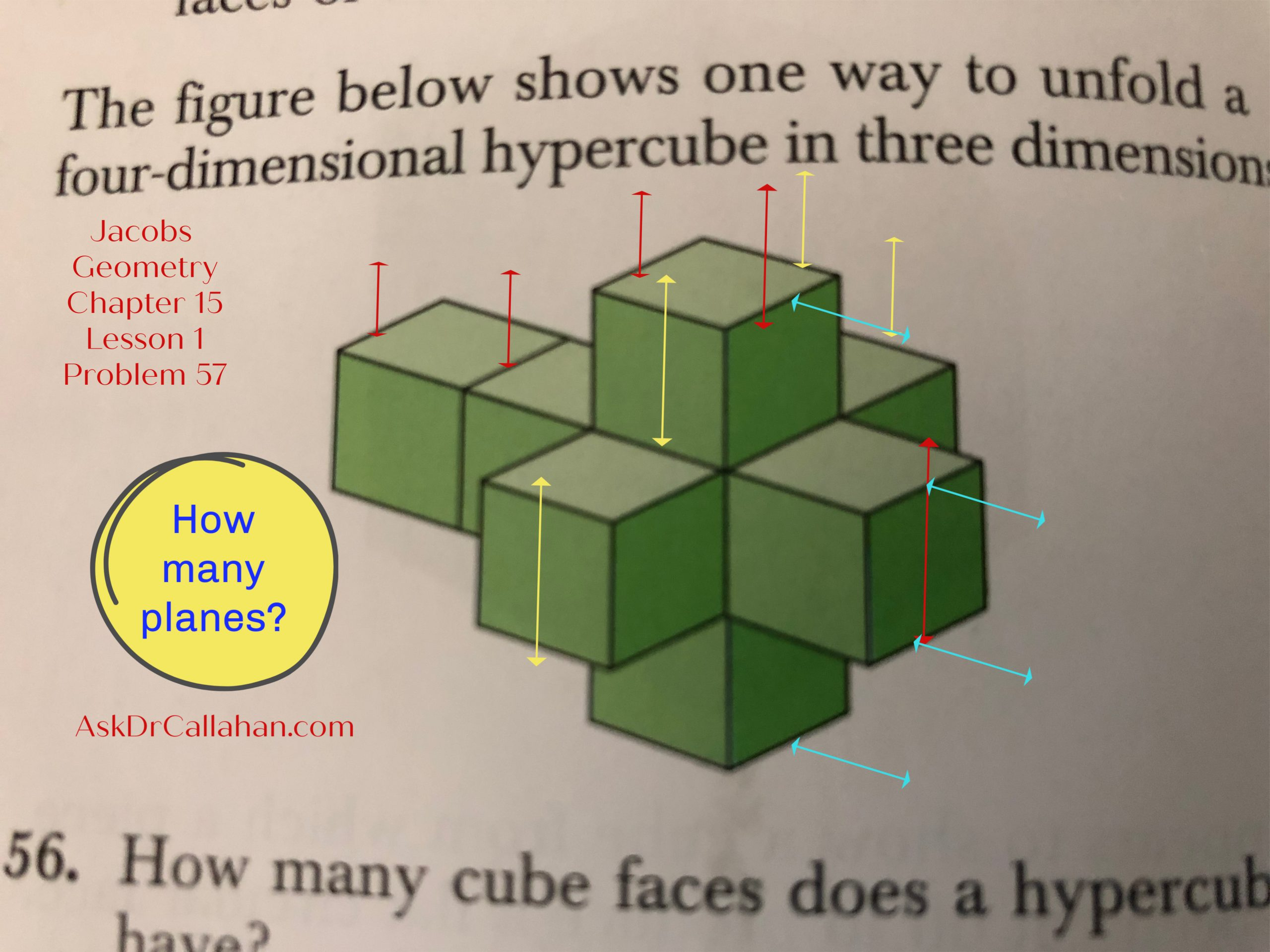In Jacobs Geometry Chapter 15 Lesson 1 problem 57, we are asked,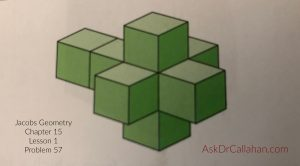“In how many planes do the square faces of the cube faces of the unfolded hypercube lie?”

The Solutions Manual says 13 planes. Let’s see how they got that number.

Imagine the red, yellow, and blue lines drawn on the hypercube as planes.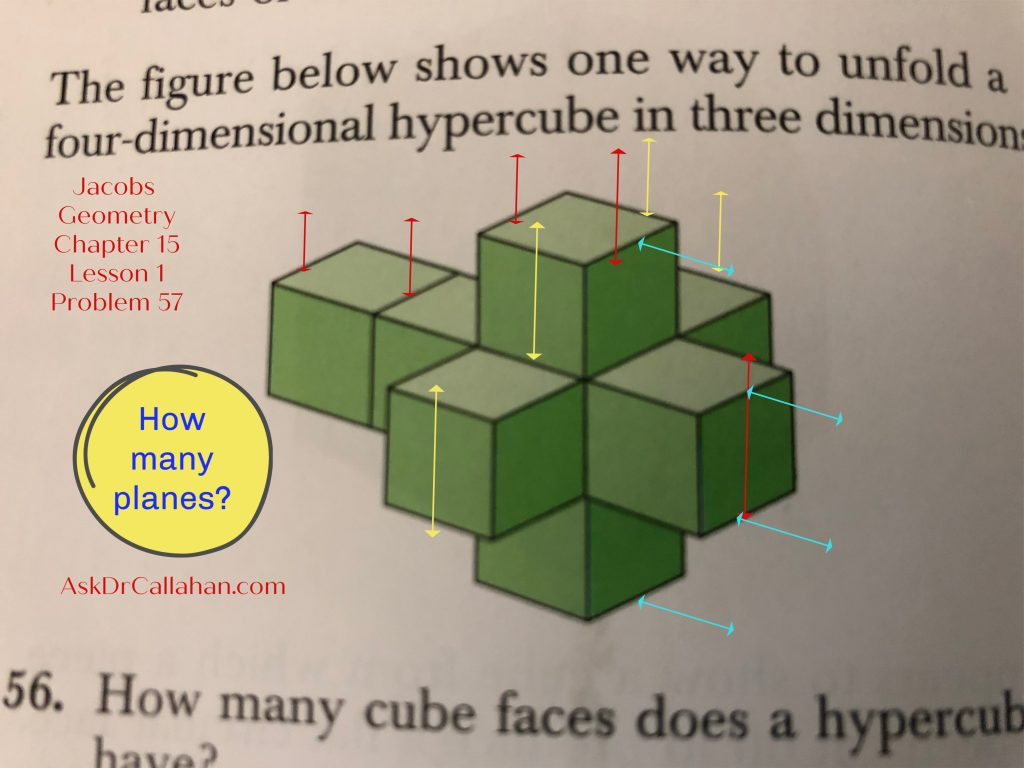Counting vertical planes from right to left: marked in red we have 5.

Counting vertical planes from front to back: marked in yellow we have 4.

Counting horizontal planes from bottom to top: marked in blue we have 4.

5 + 4 + 4 = 13 planes.

Need help with your homework problem? AskDrCallahan video instruction students can submit questions to support@askdrcallahan.com or through our website form on the student help page. Please always include the course, chapter, lesson, and problem number. Any description of your struggle is also very helpful so we get directly to your issue.  We love to help.

Posted on

## Geometry: Note on Missing Chapters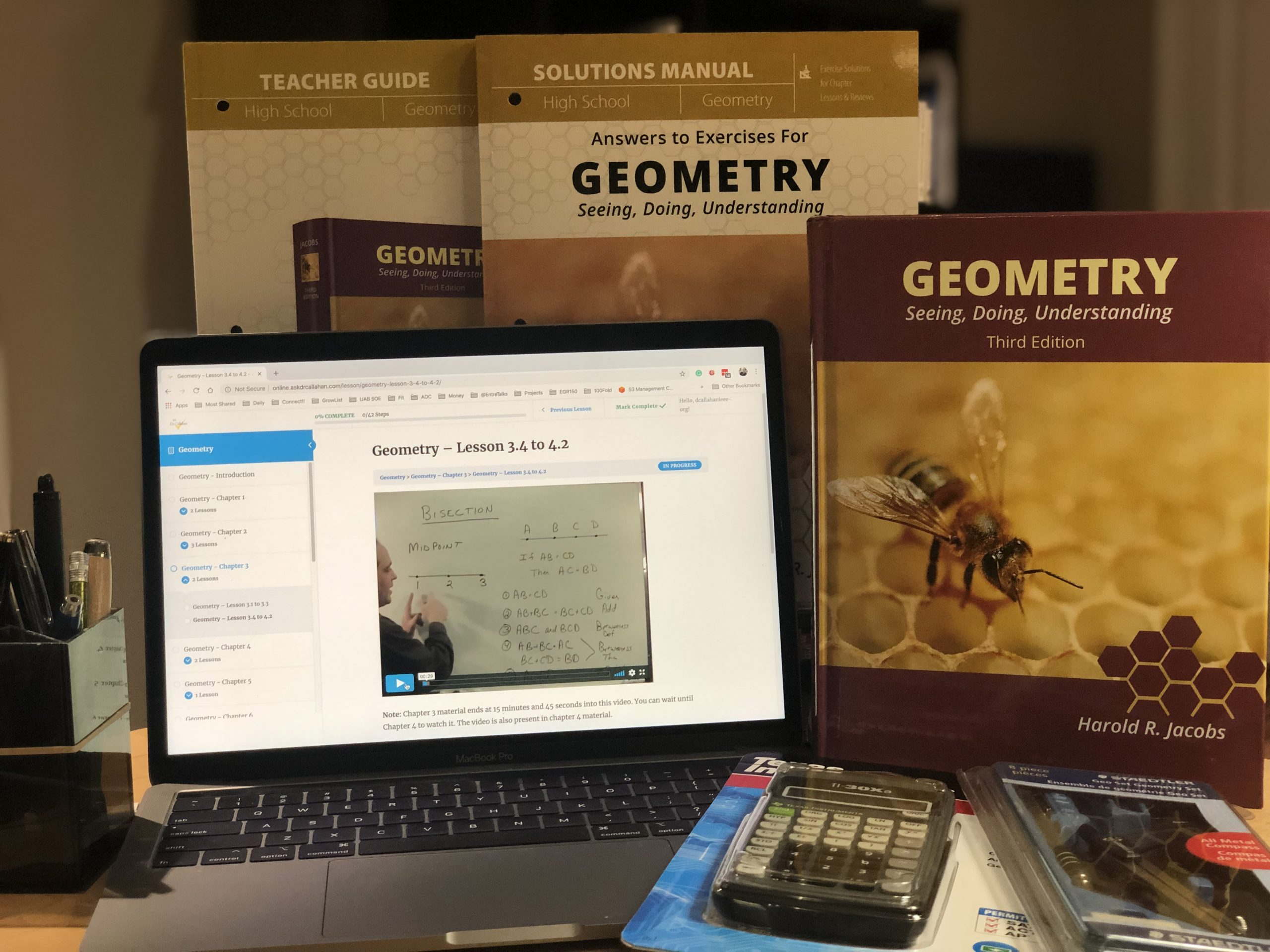## Geometry Course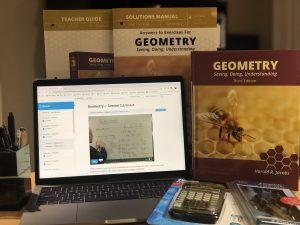Chapters 13 and 16 were originally omitted from our Geometry course for time constraints. The concepts in those chapters are advanced, though not difficult. Students will not likely see them on the ACT/SAT or even in college, except for specialized fields. When needed in a college track, they will be covered anew. They are often skipped at the high school level.

## Geometry Chapter 13 and Chapter 16However, we now recommend Chapter 13 be covered. Chapter 14 briefly refers to concepts introduced there and some students need the information. Others will be okay just referring back. Most of our students still skip chapter 13. We still consider Chapter 16 optional for everyone.
We have lectures for both chapters now on our website for FREE.
Remember that our video instruction, online or DVD, can be used with any printing of the 3rd Edition of Jacobs Geometry.
Posted on

## Homework Help Complex Problems – Moving Deeper into Concepts

As you move deeper into each textbook (Algebra, Geometry, Calculus, etc), the problems will contain several concepts you’ve learned earlier – all in one problem.  This complexity can make it difficult to find the “how-to” or examples in the book to help us understand how to work it.

Recently, we received this question from our student support page: There doesn’t seem to be any examples or teaching that I can find that helps me solve this problem.

This is a common question – not this particular problem, but in general, as the problems develop into including several simple concepts stacked into compound calculations. However, the explanations are there, they just may be back a few, or several, chapters.

For example, Jacobs Algebra Chapter 12 Summary and Review Problem 14h.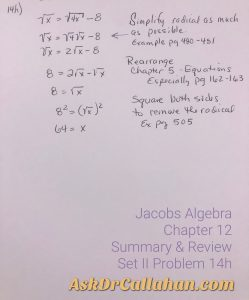Concepts include but are not limited to:

• Chapter 12: Square Roots. Simplify radical as much as possible. Example of this step page 480-481
• Chapter 5: Equations in One Variable. Specifically for this review problem, Equivalent Equations (Lesson 3) page 162-163.
• Chapter 12: Square Roots: Radical Equations. Page 505 has examples of squaring both sides to eliminate the radical.

Math builds on itself. As you learn more and more concepts, the problems reach back and build on calculating and analyzing skills learned earlier in the book or even in an earlier course. These complex problems can be hard to find “how-to” when we just can’t see it! The solutions manual is a good resource to help with steps, but sometimes even with those steps, we need to see where it was explained or taught.

We are here to help! Send us your homework questions and let us help. Filling out this form makes it easy to be sure you’ve told us what we need to know to help you, but you can also send an email to support@askdrcallahan.com.

## Be sure to tell us:

1. the course,
2. the chapter,
3. the lesson,
4. the problem,
5. YOUR issue as best as you can explain it.

We love to help.

Posted on

## Algebra Chapter 16 Lesson 2 Set 1 Problem #3

This is an error in the book by Master Books (ISBN: 978-0890519851) – the book with the blue cover showing the world.

The problem is discussing hens and rabbits with a total of 30 heads and 86 feet.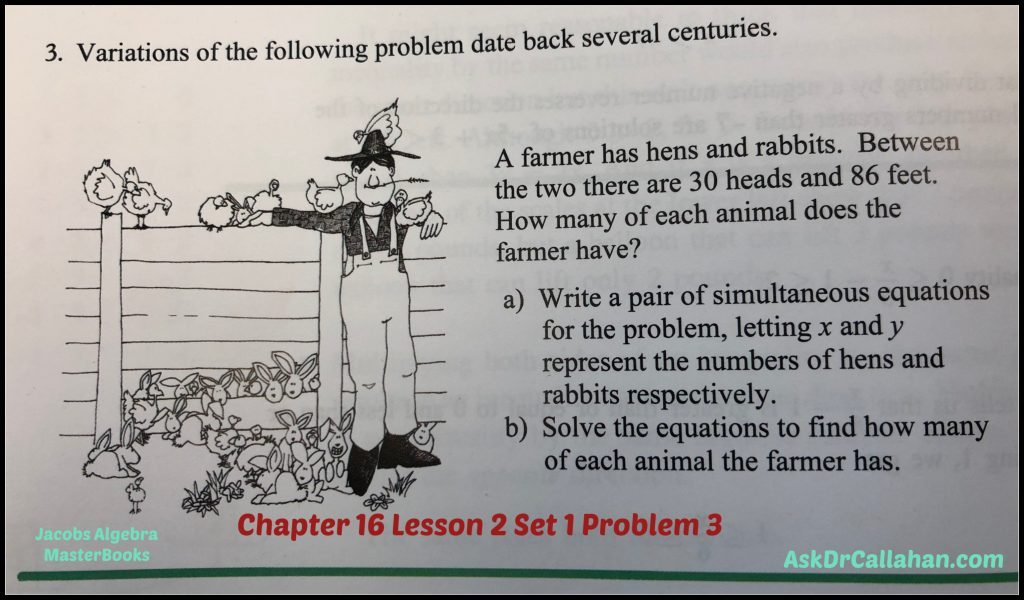ERROR: The solution manual is referring to the wrong problem which is related to money.

Here is how to solve. Simultaneous equations are covered in Chapter 7. So if you forget how to solve these, refer back to chapter 7.

heads = # hens + # rabbits

feet = 2 x # hens + 4x number of rabbits

So let h be the number of hens and r be the number of rabbits.

We can re-write the above as

heads = 30 = h + r

feet = 86 = 2h + 4r

30=h+r

So r = 30-h

Substitute this equation into the second equation to get

86 = 2h + 4r

86 = 2h + 4(30-h) = 2h + 120 – 4h = -2h +120

Rearrange to get

2h = 120-86 = 34

h = 17 (number of hens)

Now we can find the rabbits are

R = 30-h = 30-17 = 13

Checking our work

13 + 17 = 30 (total number of heads)

2 x 17 + 4 x 13 = 86 which are the number of feet.

Correct!

Posted on

## Algebra Ch13 Test A Problem #13

Question from Grace:

We are using this test A as a practice test. We can’t figure out how to solve this.

Grace,

To solve this one, you take the square root of each side

+/- SQRT ( 16x^2 + 8x +1 ) = +/- SQRT (9)

The left side needs a little work to do this. Best to see if we can factor
16x^2 + 8x +1

As a hint, you know your factors would need to be the same since you are taking a square root.

So start with the first term 16x^2 – square root of it is 4x. So try that

(4x + ___) (4x + ___)

Then the last term is 1. So the obvious thing that can be multiplied by itself to get 1 is 1. So try

(4x + 1) (4x + 1)

Now when I multiply is out I get

16x^2 + 4x + 4x + 1 = 16x^2 + 8x +1

So that works!!

Now back to the problem

+/- SQRT ( 16x^2 + 8x +1 ) = +/- SQRT (9)

+/- SQRT ((4x + 1) (4x + 1)) = +/- SQRT (9)

Using the + we have
(4x + 1) = 3
And 4x = 2 so x = 1/2 is one answer

Using the – we have
(4x + 1) = -3
4x+1 = -3
4x = -4
x = -1

Posted on

## Algebra Ch10.2 #3Question from Makenna

I don’t understand how the perimeter can be 678 ft.
255 is the width x 2 = 510
505 is the length x 2 = 1010 + 510 = 1520

Makenna,

You are correct. The 678 was from the older version of the book (which used a flag of 104 x 235. Flags have grown since then. 😉)

Just FYI – the older flag was flown in Detroit on the side of the J.L. Hudson Store – which no longer exists.

Posted on

## Geometry Ch11 test #7b

Question from Anya
Course: Geometry
Chapter/Lesson/Problem11 test7b
Issue: I’m supposed to find Angle APM. To find the angle, I used tan APM=20/70, which gives 15.9 degrees. The solutions manual used sin APM=20/70, which gives 16.6 degrees. Sine is opposite/hypotenuse. However, it seems to me that the given measurements of the problem are opposite side (20) to the adjacent side (70). Which is correct? Thank you!
Yes, you are correct. That should be tangent as you stated.
tan(APM) = 20/70
Posted on

## Algebra Ch5.6 #15

Ch5.6 #15

As before – all are similar so I will pick the harder one.

Givens

AB = CD

AB = 9(x-2)

CD = x + 3(x+5)

Therefore,

9(x-2) = x + 3(x+5)

Now multiply out and solve

9x – 18 = x + 3x + 15

9x – x – 3x  = 15 + 18

5x = 33

x = 6.6

Now go back and find the lengths

AB = 9(x-2) = 9(6.6 – 2) = 41.4

CD = AB = 41.4

CE = x = 6.6

ED = 3(x+5) = 3(6.6+5) = 34.8